Start typing, then use the up and down arrows to select an option from the list.# Weak Base Strong Acid Titration Practice

Jules Bruno
181views
2
calculate the pH of the solution that results from the mixing of 75 MLS of 750. Mueller sodium acetate and 75 MLS of 750.150 Mueller acetic acid with 0.25 Mueller of hydroponic acid. Here we're told, the K of acetic acid is 1.8 times 10 to the negative five. So we have acetic acid here based on the rules that we know for oxy acids, we know it's a weak acid, and even if you don't remember those rules again because it's K is less than one, it is a weak acid. So we have a weak acid, we have its conjugate base and we have again a strong acid. Here we have weak and strong species reacting. Therefore, we're gonna do a nice CF chart. Whatever strong has to be a reactant. And here if since the strong acid is going to react with a conjugate base, it reacts with its chemical opposite, which is a base now, remember again, what is an acid do and acid donates H plus over to the base. So we're gonna get here. As a result, arsenic acid being formed plus an a b r. We have a nice CF chart. So that's initial change. Final? Yeah, we only care about the weak acid, its conjugate base and whatever strong the fourth species we ignored. Now remember, we need moles which is just leaders times molar ity. So divide this by 1000 and divide this by 1000. Multiply them by their polarities to get the moles hbr We already have it in moles so we don't have to do any types of conversion. Just plug it right in here for the conjugate base, it'll be 0.75 moles and then for the weak acid, it's 0.1125 moles look at the react inside Now the smaller moles will subtract from the larger moles. So at the end we'll have 0.50 zero Here on the product side, we add because of the conservation of mass Now we look at the end and see what we have. At the end of this we have conjugate base remaining. We have weak acid. Remaining conjugate base plus weak acid means we have a buffer. Therefore we use the Henderson Hasselbach equation, So pH equals P K plus log of conjugate base over weak acid. Remember, P K is just the negative log of K A plus log of conjugate base amount, which is 0.50 moles of sodium acetate divided by 0.1375 moles of acetic acid when we plugged that in this part is gonna give me 4. Plus, when we take the log of everything in here that's gonna give me negative 0.4393 added together, it's gonna give me 4.31 as my final pH. So remember, this one is a bit different from the one we saw earlier, because here we still have a buffer present. As a result, we use the Henderson Hasselbach equation. Remember, we have a buffer present before you reach the equivalence point in our acid based hi, Trish. In therefore, use the Henderson Hasselbach equation to get our final answer. So remember all the steps necessary when we're dealing with a three spots in a tight trey Shen is a before an equivalence point after an equivalence point or at the equivalence point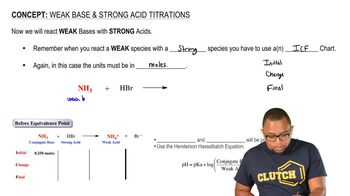01:49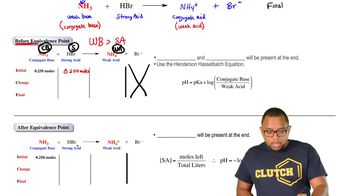02:15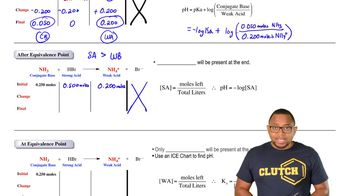01:39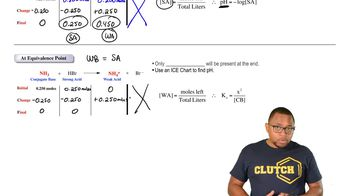01:41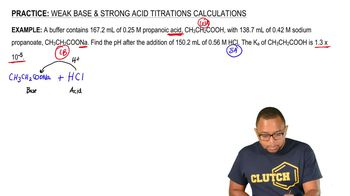05:37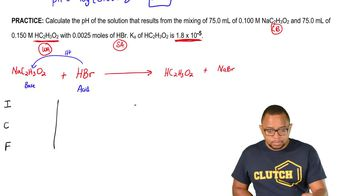04:19